Prisma修图软件的图片风格转换算法 顶原

（来自个人微信公众号：deeplearningdigest）

1.图片风格转换模型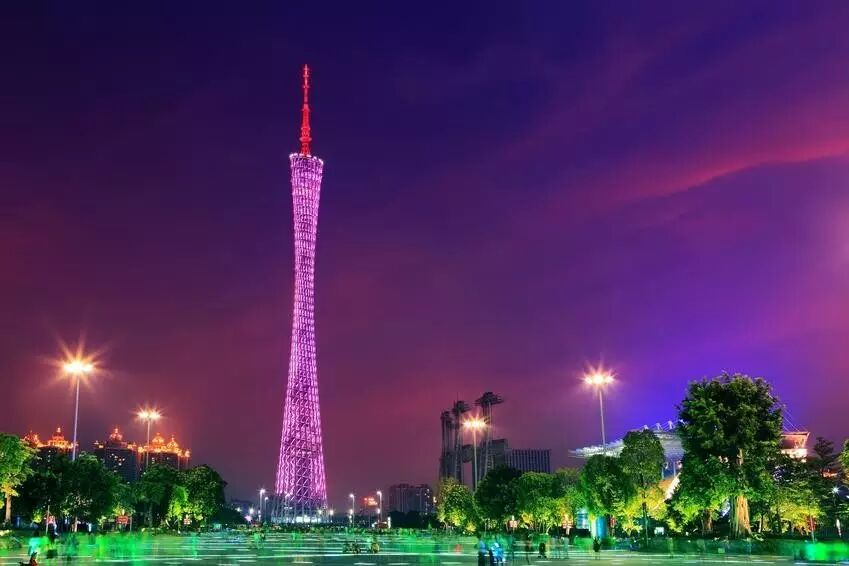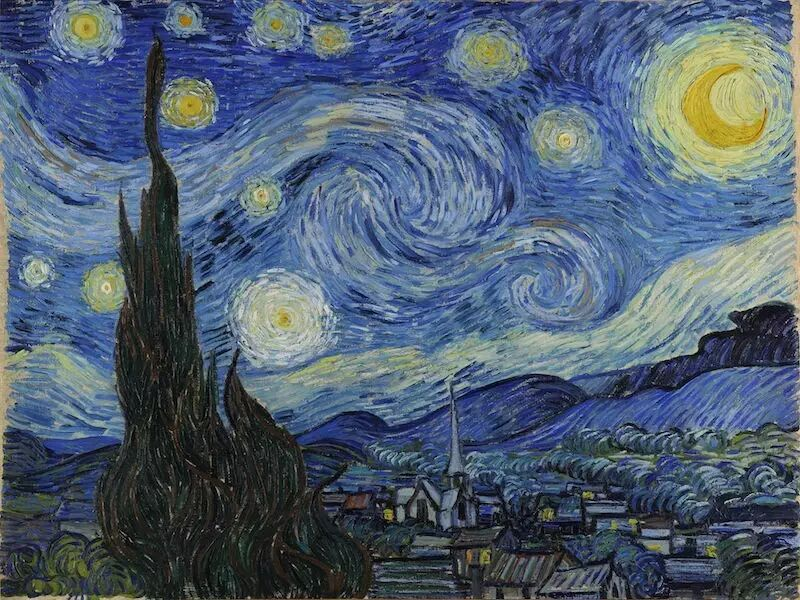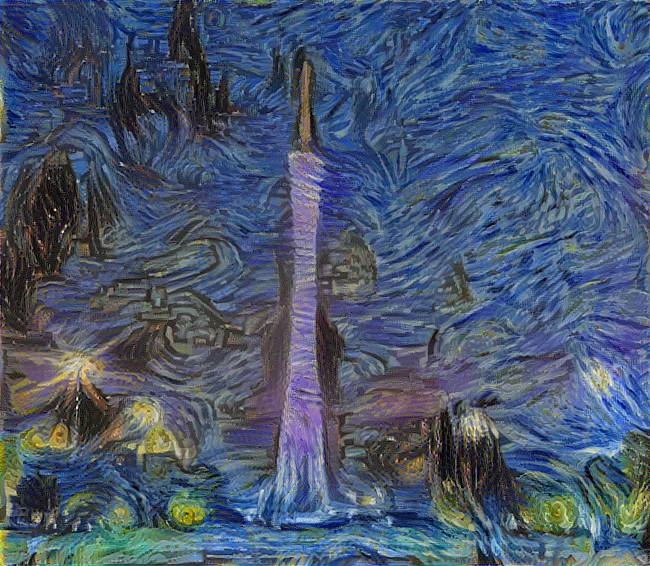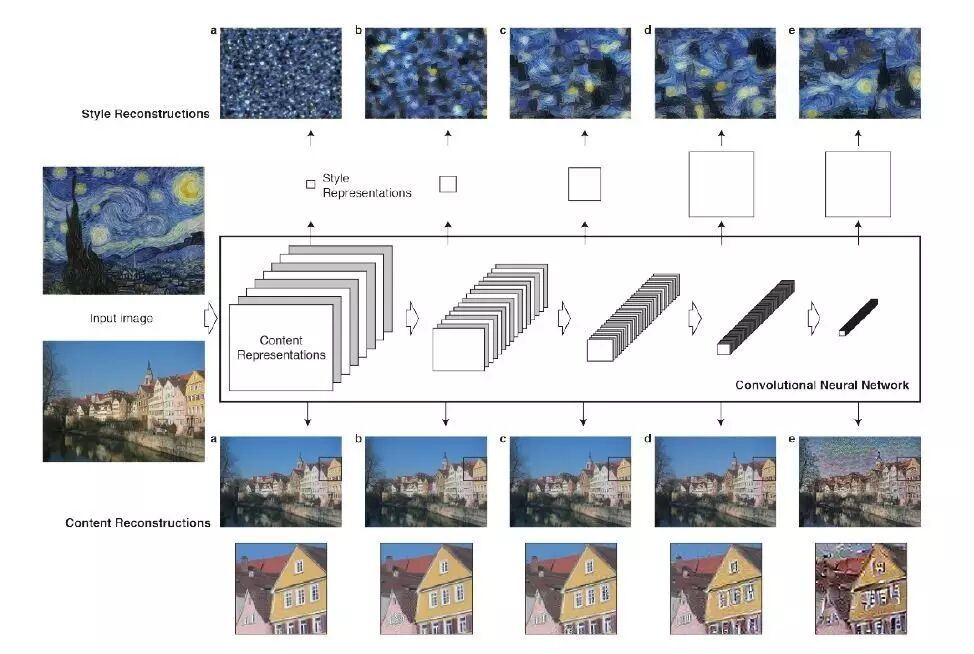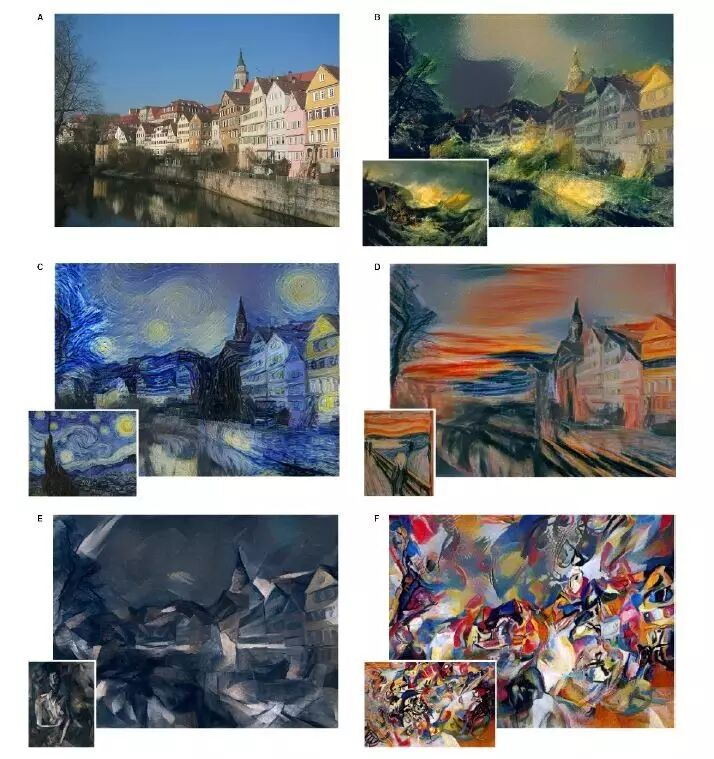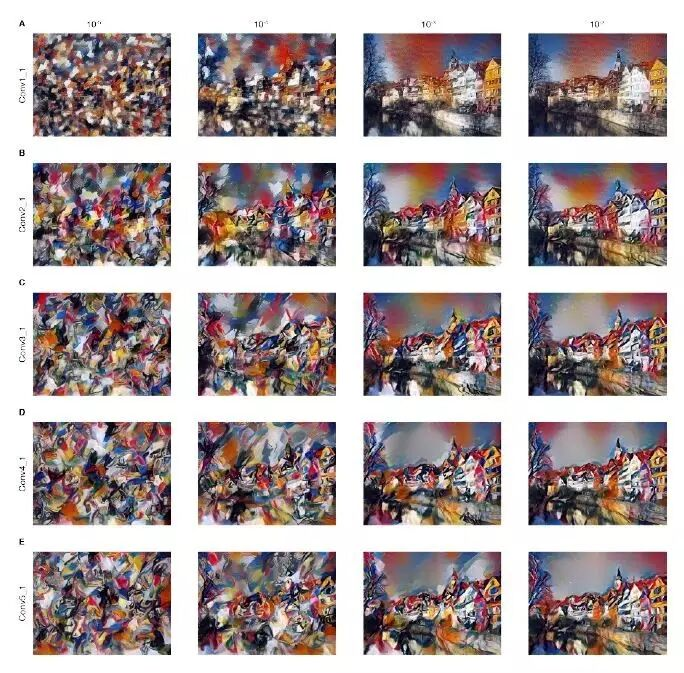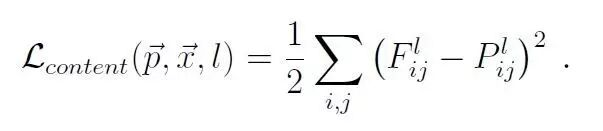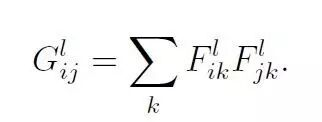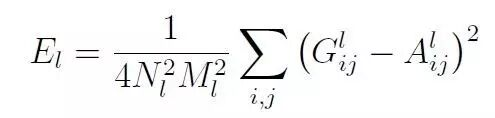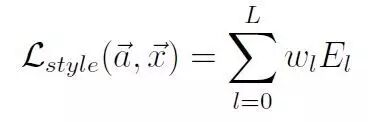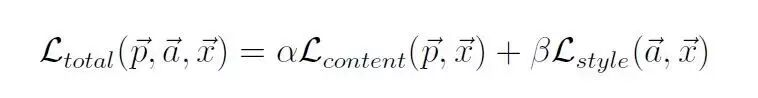2.使用TensorFlow实现模型

import tensorflow as tf

import numpy as np

import scipy.io

import scipy.misc

import os

IMAGE_W = 650

IMAGE_H = 566

#内容图片

CONTENT_IMG =  './images/15.jpg'

#风格图片

STYLE_IMG = './images/StarryNight.jpg'

#输出目录

OUTOUT_DIR = './results5'

OUTPUT_IMG = 'results.png'

#预训练的VGG19层深度卷积神经网络模型

VGG_MODEL = 'imagenet-vgg-verydeep-19.mat'

#噪声比例为0.7

INI_NOISE_RATIO = 0.7

#风格强度为500

STYLE_STRENGTH = 500

ITERATION = 5000

#内容层

CONTENT_LAYERS =[('conv4_2',1.)]

#风格层

STYLE_LAYERS=[('conv1_1',1.),('conv2_1',1.5),('conv3_1',2.),('conv4_1',2.5),('conv5_1',3.)]

MEAN_VALUES = np.array([123, 117, 104]).reshape((1,1,1,3))

def build_net(ntype, nin, nwb=None):

if ntype == 'conv':

return tf.nn.relu(tf.nn.conv2d(nin, nwb, strides=[1, 1, 1, 1], padding='SAME')+ nwb)

elif ntype == 'pool':

return tf.nn.avg_pool(nin, ksize=[1, 2, 2, 1],

strides=[1, 2, 2, 1], padding='SAME')

def get_weight_bias(vgg_layers, i,):

weights = vgg_layers[i]

weights = tf.constant(weights)

bias = vgg_layers[i]

bias = tf.constant(np.reshape(bias, (bias.size)))

return weights, bias

def build_vgg19(path):

net = {}

vgg_layers = vgg_rawnet['layers']

net['input'] = tf.Variable(np.zeros((1, IMAGE_H, IMAGE_W, 3)).astype('float32'))

net['conv1_1'] = build_net('conv',net['input'],get_weight_bias(vgg_layers,0))

net['conv1_2'] = build_net('conv',net['conv1_1'],get_weight_bias(vgg_layers,2))

net['pool1']   = build_net('pool',net['conv1_2'])

net['conv2_1'] = build_net('conv',net['pool1'],get_weight_bias(vgg_layers,5))

net['conv2_2'] = build_net('conv',net['conv2_1'],get_weight_bias(vgg_layers,7))

net['pool2']   = build_net('pool',net['conv2_2'])

net['conv3_1'] = build_net('conv',net['pool2'],get_weight_bias(vgg_layers,10))

net['conv3_2'] = build_net('conv',net['conv3_1'],get_weight_bias(vgg_layers,12))

net['conv3_3'] = build_net('conv',net['conv3_2'],get_weight_bias(vgg_layers,14))

net['conv3_4'] = build_net('conv',net['conv3_3'],get_weight_bias(vgg_layers,16))

net['pool3']   = build_net('pool',net['conv3_4'])

net['conv4_1'] = build_net('conv',net['pool3'],get_weight_bias(vgg_layers,19))

net['conv4_2'] = build_net('conv',net['conv4_1'],get_weight_bias(vgg_layers,21))

net['conv4_3'] = build_net('conv',net['conv4_2'],get_weight_bias(vgg_layers,23))

net['conv4_4'] = build_net('conv',net['conv4_3'],get_weight_bias(vgg_layers,25))

net['pool4']   = build_net('pool',net['conv4_4'])

net['conv5_1'] = build_net('conv',net['pool4'],get_weight_bias(vgg_layers,28))

net['conv5_2'] = build_net('conv',net['conv5_1'],get_weight_bias(vgg_layers,30))

net['conv5_3'] = build_net('conv',net['conv5_2'],get_weight_bias(vgg_layers,32))

net['conv5_4'] = build_net('conv',net['conv5_3'],get_weight_bias(vgg_layers,34))

net['pool5']   = build_net('pool',net['conv5_4'])

return net

def build_content_loss(p, x):

M = p.shape*p.shape

N = p.shape

loss = (1./(2* N**0.5 * M**0.5 )) * tf.reduce_sum(tf.pow((x - p),2))

return loss

def gram_matrix(x, area, depth):

x1 = tf.reshape(x,(area,depth))

g = tf.matmul(tf.transpose(x1), x1)

return g

def build_style_loss(a, x):

#M为面积。N为深度。

M = a.shape*a.shape

N = a.shape

A = gram_matrix_val(a, M, N )

G = gram_matrix(x, M, N )

loss = (1./(4 * N**2 * M**2)) * tf.reduce_sum(tf.pow((G - A),2))

return loss

image = image[np.newaxis,:IMAGE_H,:IMAGE_W,:]

image = image - MEAN_VALUES

return image

def write_image(path, image):

image = image + MEAN_VALUES

image = image

image = np.clip(image, 0, 255).astype('uint8')

scipy.misc.imsave(path, image)

def main():

net = build_vgg19(VGG_MODEL)

sess = tf.Session()

sess.run(tf.initialize_all_variables())

#产生一张白噪声图片

noise_img = np.random.uniform(-20, 20, (1, IMAGE_H, IMAGE_W, 3)).astype('float32')

#把输入赋给content_img

sess.run([net['input'].assign(content_img)])

cost_content = sum(map(lambda l,: l*build_content_loss(sess.run(net[l]) ,  net[l])

, CONTENT_LAYERS))

sess.run([net['input'].assign(style_img)])

cost_style = sum(map(lambda l: l*build_style_loss(sess.run(net[l]) ,  net[l])

, STYLE_LAYERS))

cost_total = cost_content + STYLE_STRENGTH * cost_style

train = optimizer.minimize(cost_total)

sess.run(tf.initialize_all_variables())

sess.run(net['input'].assign( INI_NOISE_RATIO* noise_img + (1.-INI_NOISE_RATIO) * content_img))

if not os.path.exists(OUTOUT_DIR):

os.mkdir(OUTOUT_DIR)

for i in range(ITERATION):

sess.run(train)

if i%2 ==0:

result_img = sess.run(net['input'])

print sess.run(cost_total)

write_image(os.path.join(OUTOUT_DIR,'%s.png'%(str(i).zfill(4))),result_img)

write_image(os.path.join(OUTOUT_DIR,OUTPUT_IMG),result_img)

3.实验结果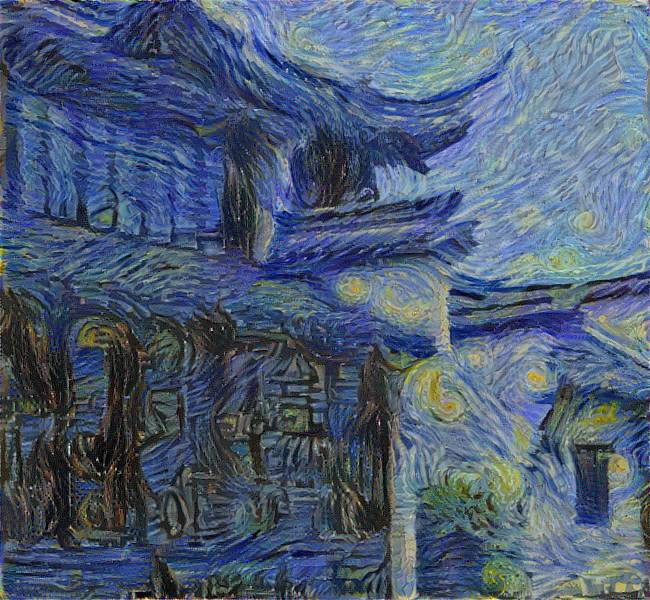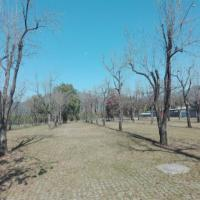断桥残雪断桥残雪

2017/12/13
0
0

dany_dai
2016/10/14
682
0

2016/12/02
590
2

Efackw13
2017/05/28
0
0

2017/08/09
0
0

lianbang_W
22分钟前
2
0

30分钟前
2
0

<!DOCTYPE html><html><head> <meta http-equiv="Content-Type" content="text/html; charset=utf-8" /> <meta name="viewport" content="initial-scale=1.0, user-scalable=n......

32分钟前
4
0

[TOC] 1.1 基本语法：插值表达式 <template> <div> {{username}} <br/> {{1+2+3}} <br/> {{'你的名字是：' + username}} <br/> {{'abc'.split('')}} </div><......

3
0
CentOS Linux 7上将ISO映像文件写成可启动U盘

4
0